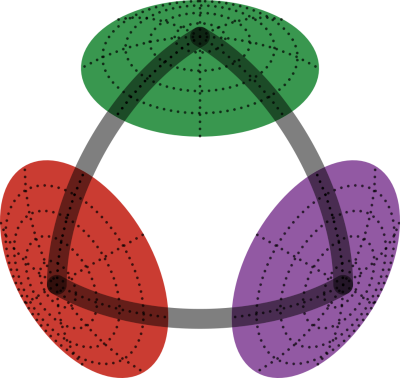# Manifolds.jl

Manifolds.jl provides a library of manifolds based on the ManifoldsBase.jl interface to get an easy start to use manifolds within you project. It aims to provide a simple and efficient implementation of all necessary functions on manifolds.

## References

1. AxenBaranBergmannRzecki-2021Axen, S. D., Baran, M., Bergmann, R., Rzecki, K.2021Manifolds.jl: An Extensible Julia Framework for Data Analysis on Manifolds
arXiv 2106.08777

For data given on a nonlinear space, like angles, symmetric positive matrices, the sphere, or the hyperbolic space, there is often enough structure to form a Riemannian manifold. We present the Julia package Manifolds.jl, providing a fast and easy to use library of Riemannian manifolds and Lie groups. We introduce a common interface, available in ManifoldsBase.jl, with which new manifolds, applications, and algorithms can be implemented. We demonstrate the utility of Manifolds.jl using Bézier splines, an optimization task on manifolds, and a principal component analysis on nonlinear data. In a benchmark, Manifolds.jl outperforms existing packages in Matlab or Python by several orders of magnitude and is about twice as fast as a comparable package implemented in C++.

@online{AxenBaranBergmannRzecki-2021, eprint = {2106.08777}, author = {Axen, S. D. and Baran, M. and Bergmann, R. and Rzecki, K.}, year = {2021}, eprinttype = {arXiv}, title = {Manifolds.jl: An Extensible Julia Framework for Data Analysis on Manifolds}, abstract = { For data given on a nonlinear space, like angles, symmetric positive matrices, the sphere, or the hyperbolic space, there is often enough structure to form a Riemannian manifold. We present the Julia package Manifolds.jl, providing a fast and easy to use library of Riemannian manifolds and Lie groups. We introduce a common interface, available in ManifoldsBase.jl, with which new manifolds, applications, and algorithms can be implemented. We demonstrate the utility of Manifolds.jl using Bézier splines, an optimization task on manifolds, and a principal component analysis on nonlinear data. In a benchmark, Manifolds.jl outperforms existing packages in Matlab or Python by several orders of magnitude and is about twice as fast as a comparable package implemented in C++. }, }